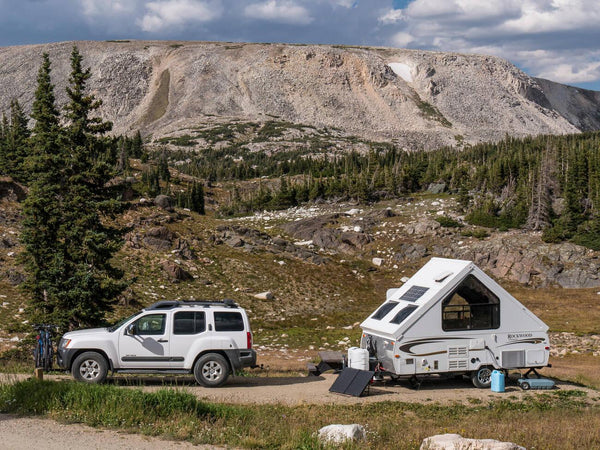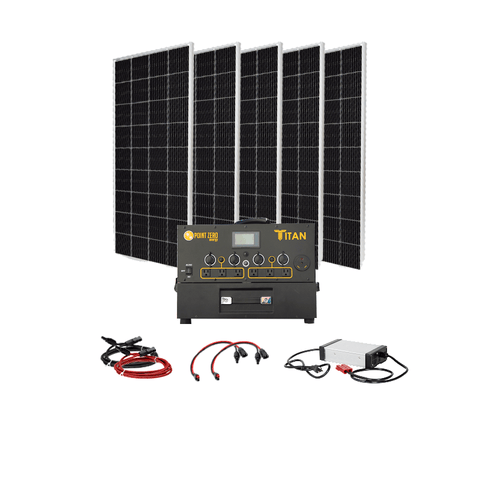RV Amp Hour Calculator - Guide & Step-by-Step Tutorial - ShopSolar.com

# RV Amp Hour Calculator

When you’re starting up your RV solar journey, you’re going to need to understand a few concepts about battery sizing and capacity. One of these concepts is amp hours.

Let’s go over how to use an amp hour calculator as well as understand the measurement itself.

## Amp Hours

Amp-hours are often associated with batteries. It’s the amount of energy charged in a storm that allows 1A to flow for 1 hour.

You may find that some amp calculators for houses return an amp hour value as an average of how many amps are needed in your home.But understanding this measurement of power means we can

When you see a battery on the store shelf, it will say 100Ah at 13V as a power rating. But what does that mean? Let’s use this battery more practically.

Let’s say we have two of these batteries that are connected in parallel.

Batteries are like solar panels in that their amp hours get added together while the voltage remains the same when connected to a system.

If these batteries are wired in series to the system, the voltages are added together while the amp hours remain the same.

Neither set has more capacity despite the one operating at more amp hours than the other. But this is where the amp hour calculator comes into play.

Before we visit the formula, you must have the necessary values. For example, convert volts to amps, or ohms to amps using the ohms law.

## The Amp-Hour Formula

The amp hours formula states that the amps multiplied by the volts are equivalent to the watts. This also means that the amp hours multiplied by the volts equals the watt-hours.So let’s use practical values here. in the first setup when our batteries were connected in parallel, the watt hours were equivalent to 2,600Wh, and the second set is 2,600Wh or 2.6KWh.

This ease of calculation makes using an Ah to kWh calculator a breeze.

Let’s use a lightbulb for an RV as an example.

If we have a lightbulb in our RV solar setup rated at 80W, and it's being powered for 1 hour, then it consumes 80Wh.

This formula can be used to determine how much power is being consumed by a particular load.

But how does this relate to amp hours?

If we have a battery rated at 100Ah at 13V and it's powering an 80W RV lightbulb. We want to find out how many amps are flowing along the wires connected to the light bulb.

We could use a VA to amps calculator but for the sake of today’s topic, we’re going to use an RV amp hour calculator.

For this, we need to use the watts as equivalent to the amps multiplied by the volts. So once all the multiplication is done, we get around 7.69A. This means that there’s 7.59A running along the wires.

This means that if we run the lightbulb for 1 hour, it would pull 7.69A amp hours from the battery bank connected to the RV’s solar system.

If we want to increase the voltage of the battery, the formula remains the same but the overall amp hours would be less.

However, this doesn’t mean that one setup is pulling more power than the other. The lightbulb is still 80W, the 80W is being delivered at a different voltage making the amperage different.

But what does the voltage difference mean?

### The voltage differences

Let’s say we keep the 100Ah at 13V battery but include the inverter, the heater, and our array of flexible solar panels for our RV solar system.The inverter is rated at 1,200W but you can use an inverter amp draw calculator to get a reading suited to your RV solar setup.

If we power our heater directly from the battery, we will end up with a value of around 92A flowing to the heater.

Now let’s incorporate the inverter into our equation. We want to connect the battery to our inverter and the inverter to our heater.

The inverter takes 12V from the battery and converts it to 120V for the heater.

Both sides are still pulling 1,200W, so one side will have 92A flowing while the other is around 10A. the side where the inverter is connected to the heater has a significantly lower amperage compared to the battery side.

From this, we can say that the amp hours used from a battery bank should be calculated from the battery bank’s operating voltage.

But how do we apply what we now know about amp hours for our RV solar system?

### Calculating Amp-Hours for Your RV

The first step is to read the labels on the appliances you want to power using your RV’s solar system. This tends to be rated in volts and current.

The next step is to retrieve the power consumption for each of the appliances. This is done by calculating the watts using ohms or watt’s law based on the values you have.Now multiply this value by the number of hours that they’d be running to obtain your watt-hours. Add up all these values to get the total number of watt-hours used by all appliances.

Converting to amp hours is simply a matter of taking the watt hours and dividing it by the voltage.

## Conclusion

Knowing how to properly size your RV’s solar system is a good way to prepare yourself for long road trips or adventures.

Amp-hours, watt-hours, volts, and resistance are all interchangeable through various laws and calculators.

Did You Find Our Blog Helpful? Then Consider Checking: